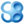# Simulation of SBML model with Mathematica using MathSBML

## What is MathSBML?

MathSBML is an open-source package for working with SBML models in Mathematica, developed by Bruce E. Shapiro. It provides facilities for reading SBML models, converting them to systems of ordinary differential equations for simulation and plotting in Mathematica, and translating the models to other formats. It supports both Level 1 and Level 2 SBML.

## Download and Installation

To download the package, including complete documentation, please visit the MathSBML area at SourceForge.net.

### 1. Download and Install MathSBML

1. First setup Mathematica.
note: We have tested CellDesigner 2.0 with Mathematica 5.1.
2. Download MathSBML from the following site:
http://sourceforge.net/project/showfiles.php?group_id=71971&package_id=80483
3. Download MathSBML-2.4.0.zip and unzip in the appropriate directory.
4. Copy "MathSBML.m" file to the following directory.
1. On Mathematica, execute the following command to find the right directory.
\$UserAddOnsDirectory [Shift]+[Return]
2. Copy MathSBML.m file to the "Application" subdirectory under the above directory.
note: For MacOSX user, the directory should be "/Users/username/library/Mathematica/Applications"
5. Shut down Mathematica, then restart it.
6. After restarting Mathematica, input the following commands to retrieve MathSBML to Mathematica.
<< MathSBML.m [Shift]+[Return]

### 2. Simulation with MathSBML1. Place an SBML file you want to simulate in the home directory (In case of MacOSX, /Users/username/ directory).

2. Input the following commands to execute the simulation.
m = SBMLRead["filename"] [Shift]+[Return]
n = SBMLNDSolve[m, 100] [Shift]+[Return]
SBMLPlot[n] [Shift]+[Return]

• SBMLNDSolve[] on the second line is the function to run the simulation.
In the above case, "100" is the simulation runtime.
If you want to simulate the time between 0 - 20, input as follows:
• n = SBMLNDSolve[m, 20] [Shift]+[Return]
• SBMLPlot[] is the function to plot.

As MathSBML can handle Events, Rules, user-defined Functions and SBML predefined Functions, it is suitable for complex simulation.About Us | Site Map | Privacy Policy | Contact Us | ©2004 The Systems Biology Institute. All rights reserved.